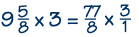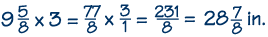Home    |    Teacher    |    Parents    |    Glossary    |    About UsMultiplying mixed numbers is just like multiplying fractions. In fact, it is multiplying fractions, since you first change the mixed numbers into improper fractions. Let's work through a "word problem" example.

Melinda is building a birdhouse and needs three pieces of wood that are each 9-5/8 inches long. She has a piece of wood that is 29 inches long. Will this be long enough?

Let's multiply 3 times 9-5/8. First we have to change both numbers to improper fractions. Three is easy; it becomes 3/1.

The next one is a little trickier. Keep the 8 in the denominator. For the numerator, multiply 9 times 8 and add 5. This gives 77 in the numerator, so the improper fraction is 77/8. This can't be simplified any further, so we go on with our multiplication.Now multiply the numerators and then the denominators. This gives 231 in the numerator, and 8 in the denominator.We want our answer in lowest terms, so we divide 231 by 8, and get 28-7/8 inches. Since this is less than 29 inches, Melinda will be able to saw her three pieces from this board, as long as she measures and cuts very carefully!Homework Help | Pre-Algebra | FractionsEmail this page to a friendSearch·  Definitions·  Reducing fractions·  Adding and subtract-     ing fractions·  Multiplying fractions·  Dividing fractions·  Adding and subtract-     ing mixed numbers·  Multiplying     mixed numbers·  Dividing     mixed numbersFirst Glance In Depth Examples WorkoutMultiplying mixed numbers